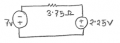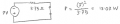# Finding Voltage Source's Contribution to Power Delivered in a Circuit (Superposition)

#### ElectronicGuru

Joined Sep 26, 2014
22
Here's the (simplified) circuit for which I have to find the power delivered by the 7 V voltage source:What I was doing was first finding the current in the circuit by combining the two voltage sources and then dividing by the 3.75 Ω resistance. From this, I used the power (supplied) equation:

Power delivered by 7 V source = ((7 v + 2.25 V) / 3.75 Ω) * (7 V) = 17.27 W.

However, the solution uses superposition to calculate the 7 V source's contribution to the power delivered:This seems wrong to me because I thought superposition could only be used for calculating linear responses, as stated in my textbook:
We must constantly be aware of the limitations of superposition. It is applicable only to linear responses, and thus the most common nonlinear response—power—is not subject to superposition.
So is the solution correct and, if so, what is my error with relation to the quote from my textbook?

#### t_n_k

Joined Mar 6, 2009
5,455
You are correct. The solution is incorrect.

#### ElectronicGuru

Joined Sep 26, 2014
22
You are correct. The solution is incorrect.
Do you know this for sure or are you basing it off what my textbook said? Just checking to be sure.

#### crutschow

Joined Mar 14, 2008
29,515
I know your first solution is correct so that means the second "solution" is not. The reason is that power is proportional to V squared so you have to take both voltages into account at the same time to calculate power. Thus 7^2 plus 2.25^2 is not equal to 9.25^2.

#### studiot

Joined Nov 9, 2007
4,998
You should not be using superposition for the power calculation, you book is quite correct.
This is due the the fact that a square (voltage or current ) is non linear.

You should, howevr note that the two batteries are in series so their voltages add.

So the total voltage applied to the resistor is (7 + 2.25) = 9.25 volts.

Ohms law provides the current I = 9.25/3.75 = 2.47 amps

Power is the product of current and voltage = VI

Now do the exercise of calculating The VI for each battery individually and adding them up and then comparing this with the power obtained by multiplying the total voltage by the current.

You might be suprised.

#### ElectronicGuru

Joined Sep 26, 2014
22
You should not be using superposition for the power calculation, you book is quite correct.
This is due the the fact that a square (voltage or current ) is non linear.

You should, howevr note that the two batteries are in series so their voltages add.

So the total voltage applied to the resistor is (7 + 2.25) = 9.25 volts.

Ohms law provides the current I = 9.25/3.75 = 2.47 amps

Power is the product of current and voltage = VI

Now do the exercise of calculating The VI for each battery individually and adding them up and then comparing this with the power obtained by multiplying the total voltage by the current.

You might be suprised.
Good tyo get confirmation. Thanks! The example you provide is actually the same one in my textbook and the reason I thought the solution was wrong.

#### studiot

Joined Nov 9, 2007
4,998
If you wish to consider this further

The reason V^2/R does not work is that neither 7 volts nor 2.25 volts is the voltage across the resistor. They are the voltages across the batteries.

The voltage across the resistor is 9.25 volts.

And for this value the formula works correctly.